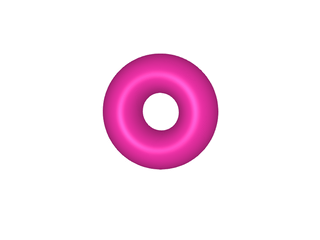# Visualization problem of the back side of a surface mesh

Hello,

I have a problem with a 3D animation. This is a torus which reverses itself. The problem is that when it is reversed, it is ugly because the normals are not oriented as they should. How to solve this problem?``````using Meshes
using MeshViz
using GLMakie
using Makie
using Printf

# parameterization of the Clifford torus
function Clifford(u, v)
[cos(u), sin(u), cos(v), sin(v)] / sqrt(2)
end

# a 4D rotation
function rotate4D(x, t)
cost = cos(t)
sint = sin(t)
[
-x*sint + x*cost,
x*cost - x*sint,
x*sint + x*cost,
-x*cost - x*sint
]
end

# modified stereographic projection
function stereog(p)
r = acos(p) / pi / sqrt(1-p*p)
(r*p, r*p, r*p)
end

# topology
nu = 300
nv = 300
topo = GridTopology((nv, nu), (true, true))

# subdivisions
u_ = LinRange(0, 2*pi, nu+1)[1:nu]
v_ = LinRange(0, 2*pi, nv+1)[1:nv]

# draw the Clifford torus
function drawCT(nplots, alpha1, alpha2)
t_ = LinRange(0, 2*pi, nplots+1)[1:nplots]
for i in 1:nplots
fig, ax, _ = viz( # invisible bounding box
Meshes.Box(Meshes.Point(-1, -1, -1), Meshes.Point(1, 1, 1));
alpha = 0
)
ax.show_axis = false
points = [
stereog(rotate4D(Clifford(u, v), t_[i]))
for u in u_ for v in v_
]
mesh = SimpleMesh(Meshes.Point.(points), topo)
viz!(mesh; color = :maroon1)
scale!(ax.scene, 1.65, 1.65, 1.65)
Makie.rotate!(fig.scene, Meshes.Vec3f(1, 0, 0), alpha1)
Makie.rotate!(ax.scene, Meshes.Vec3f(0, 0, 1), alpha2)
png = @sprintf "zzpic%03d.png" i
Makie.save(png, fig)
end
end
``````
1 Like

@ffreyer I think you fixed this in your current lighting PR? At least I think I remember some mentioning of backlighting changes and specular highlights etc.

You can fix that with `backlight = 1f0` regardless of the pr.

We could make that the default, but I’m not sure if we should. For a static surface it makes sense that only one side gets light from a single light source and with `backlight = 1f0` both sides would.

I did not know this was an option! It is not currently listed under the “3D shading attributes” when you `?mesh`. Searching the online documentation, it appears to only turn up for `band`.

Still, great to know it’s easy to get surfaces lit on both sides.

Thank you. Where should I set this option? In `viz`?